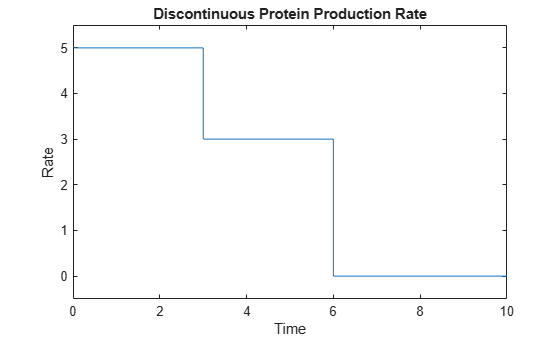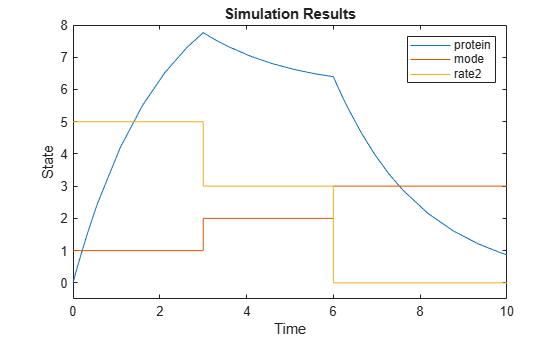# Deterministic Simulation of a Model Containing a Discontinuity

This example shows how to correctly build a SimBiology® model that contains discontinuities.

### Background

The model you create in this example simulates the first-order elimination of a protein that is produced at a specified rate. The production rate contains two discontinuities. To simulate the model accurately, you must create events that are triggered at the time of the discontinuity.

The production rate has three "modes" of production, as illustrated in the following plot:

```plot([0 3 3 6 6 10], [5 5 3 3 0 0]); ylim([-0.5 5.5]); xlabel('Time'); ylabel('Rate'); title('Discontinuous Protein Production Rate');```Initially ("Mode 1"), the production rate is a constant value of 5. From 3 to 6 seconds ("Mode 2"), the production rate is 3. After 6 seconds ("Mode 3"), the production rate is 0. These production rates are implemented in a MATLAB function discontSimBiologyRateFunction.m, which requires two arguments, simulation time and production mode.

In this example, you will add events to the model to change the mode of protein production. This approach ensures that discontinuities in the model occur only via events, which further ensures that the ODE solver accurately calculates the numerical behavior near the discontinuities.

Note that to simulate a model accurately you must use events to handle any discontinuity, whether related to function values or their derivatives.

### Construct the Model, Compartment, and Species

```model = sbiomodel('discontinuous rate'); central = addcompartment(model,'Central'); addspecies(central,'protein')```
```ans = SimBiology Species Array Index: Compartment: Name: Value: Units: 1 Central protein 0 ```

### Construct the Reaction for First-Order Elimination

`reaction1 = addreaction(model,'protein -> null')`
```reaction1 = SimBiology Reaction Array Index: Reaction: 1 protein -> null ```
```ke = addparameter(model,'ke', 0.5); kineticLaw1 = addkineticlaw(reaction1,'MassAction'); kineticLaw1.ParameterVariableNames = {ke.Name}; reaction1.ReactionRate;```

### Construct the Events That Are Triggered at the Time of Discontinuities

These events set a parameter mode that controls the mode of protein production. The mode is initially 1, changes to 2 at time 3, and changes to 3 at time 6.

```counter = addparameter(model,'mode', 1, 'ConstantValue', false); addevent(model,'time > 3', 'mode = 2')```
```ans = SimBiology Event Array Index: Trigger: EventFcns: 1 time > 3 mode = 2 ```
`addevent(model,'time > 6', 'mode = 3')`
```ans = SimBiology Event Array Index: Trigger: EventFcns: 1 time > 6 mode = 3 ```

### Construct the Reaction for Protein Production

We assign this rate to a parameter using a repeated assignment rule. This lets us store the production rate in the simulation results.

```reaction2 = addreaction(model, 'null -> protein'); rate2 = addparameter(model,'rate2', 0, 'ConstantValue', false); reaction2.ReactionRate = 'rate2'```
```reaction2 = SimBiology Reaction Array Index: Reaction: 1 null -> protein ```
`addrule(model,'rate2 = discontSimBiologyRateFunction(time, mode)', 'repeatedAssignment')`
```ans = SimBiology Rule Array Index: RuleType: Rule: 1 repeatedAssignment rate2 = discontSimBiologyRateFunction(time, mode) ```

### View the Contents of discontSimBiologyRateFunction

`type discontSimBiologyRateFunction`
```function rate = discontSimBiologyRateFunction(time, mode) %#ok<INUSL> %discontSimBiologyRateFunction - Helper function for SimBiology examples. % RATE = discontSimBiologyRateFunction(TIME, MODE); % Copyright 2010-2021 The MathWorks, Inc. % Mode is a double precision number subject to round-off errors. We need to % round to the nearest integer to correctly handle this issue. mode = round(mode); switch mode case 1 rate = 5; case 2 rate = 3; case 3 rate = 0; otherwise error('Invalid mode.'); end ```

### Simulate and Plot the Model

`model`
```model = SimBiology Model - discontinuous rate Model Components: Compartments: 1 Events: 2 Parameters: 3 Reactions: 2 Rules: 1 Species: 1 Observables: 0 ```
```sd = sbiosimulate(model); plot(sd.Time, sd.Data); ylim([-0.5 8]); xlabel('Time'); ylabel('State'); title('Simulation Results'); legend(sd.DataNames);```### Conclusion

This example illustrates how to create a SimBiology model that contains discontinuities. It illustrates how to add events to the model to address the discontinuities, so you can simulate the model accurately.